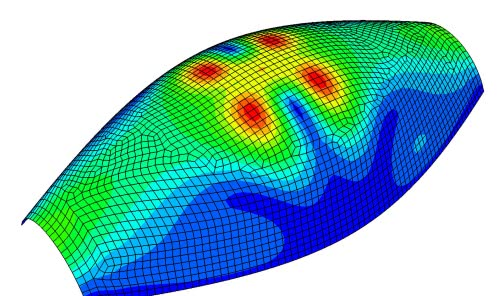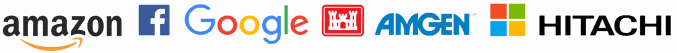# Applied functional analysis

Functional analysis is an extension of linear algebra. It’s all about linear spaces, usually spaces of functions and usually infinite dimensional. And it looks at spaces with more structure than just a vector space. In order of increasing structure there’s at least a topology (such as Fréchet spaces), often a norm (Banach spaces), and sometimes an inner product (Hilbert spaces).

## How is this practical?

At first it may seem impractical to be concerned about infinite dimensional spaces. Far from it. Infinite dimensional spaces arise naturally from studying problems that arise out of our finite world. Even when spaces are naturally finite, it can be useful to think of them being potentially infinite because this brings up important issues that we might otherwise overlook.

For example, every linear transformation on a finite space is continuous, so we can take continuity for granted. In an infinite dimensional space, linear transformations may or may not be continuous. A finite dimensional transformation is better behaved if it’s infinite dimensional analog is continuous. Otherwise it might be continuous, but it’s degree of continuity may be degrading as the dimension increases. In short, infinite dimensional spaces can guide our thinking about high-but-finite dimensional spaces.## Applications

There are many applications of functional analysis to differential equations. (I initially studied functional analysis in grad school, then segued into research in partial differential equations.) Finite element methods, for example, are easier to understand in the context of functional analysis. You formulate your problem in some infinite dimensional function space, usually a Sobolev space, and project it down to a finite dimensional approximation in terms of functions on finite elements.

The modern approach to working with partial differential equations, whether in theory or in computation, is usually framed in terms of functional analysis. The same is true of optimization.

Harmonic analysis, and applications such as signal processing, rely on stretching the limitations of classical analysis, doing things such as taking derivatives of functions that aren’t differentiable or taking the Fourier transform of functions that don’t have a Fourier transform in the classical sense. Things like the delta “function,” which isn’t really a function, come into play. All of this is justified using functional analysis. The key is to lift the context from functions acting on some space to so-called generalized functions which are linear functionals acting on some appropriate set of functions on the space. It may sound like hand-waving, but it’s all made rigorous using functional analysis.

When you have a norm on a function space, i.e a Banach space, you can do calculus on this space, often using the same calculations you could in several-variable calculus. Your norm is now a norm on an infinite dimensional space, but if you’re careful, a lot of things carry over. This is the key to the so-called calculus of variations. You can do things like taking derivatives where your variables represent functions rather than points in space. Functional analysis is critical in understanding when you can proceed on analogy with ordinary calculus and when you have to be more careful.

For help applying the tools of functional analysis to your problems, please call or email to discuss your project.

Trusted consultants to some of the world’s leading companies